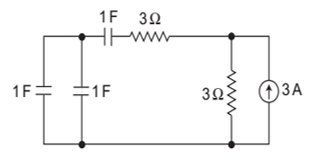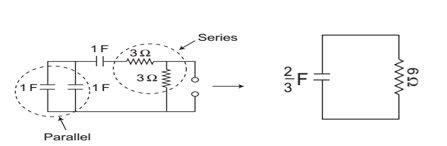Engineering Jobs   »   ELECTRICAL GATE QUIZ,

# GATE’22 EE: Daily Practices Quiz 19-July-2021

Q1-Q2 Carry 1 mark each
Negative marking: 0.33 mark

Q3-Q5 carry 2 marks each
Negative marking: 0.66 mark

Total Questions: 05
Total marks: 8
Time: 12 min.

Q1. At any power factor of the load the efficiency of a transformer will be maximum when:
(a) copper loss is equal to core loss
(b) copper loss is equal to eddy current loss
(c) copper loss is less than core loss
(d) copper loss is greater than core loss

Q2. A synchronous machine with a low value of short circuit ratio has:
(a) higher stability limit
(b) good voltage regulation
(c) good speed regulation
(d) lower stability limit

Q3. Power consumed by a balanced 3-phase; 3-wire load is measured by two wattmeter methods. The first wattmeter reads twice that of the second. Then what will be the load impedance angle in radian?
(a) (π/6)
(b) (π/3)
(c) (π/2)
(d) (π/4)

Q4. A dc shunt generator when driven without connecting field winding shows an open circuit terminal voltage of 12 V. When field winding is connected and excited the terminal voltage drops to zero because
(a) field resistance is higher than critical resistance
(b) there is no residual magnetism in the field circuit
(c) field winding has got wrongly connected
(d) there is a fault in the armature circuit

Q5. The time constant for the given circuit will be(a) 1/9 s
(b) 1/4 s
(c) 4s
(d) 9s

SOLUTIONS

S1. Ans.(a)
Sol. At any power factor of the load, the efficiency of a transformer will be maximum when the copper loss is equal to core loss.

S2. Ans.(d)
Sol. X_d in p.u = 1/SCR
SCR =1/X_d in per unit
Thus, short circuit ratio is equal to the reciprocal of per unit value of direct axis synchronous reactance. The more the value of Xd, the lesser will be the short circuit ratio.
For the small value of the short circuit ratio (SCR), the synchronizing power is small. As the synchronizing power keeps the machine in synchronism, a lower value of the SCR has a low stability limit. In other words, a machine with a low SCR is less stable when operating in parallel with the other generators.

S3. Ans.(a)
Sol. W_1=2W_2
W_(1 ):W_2=2:1
Let W_1=2
W_2=1
Then,
tanθ= √3 ((W_1-W_2)/(W_1+W_2 ))
=√3 ((2-1)/(2+1))
=√3×1/3=1/√3

S4. Ans.(c)
Sol. The wrongly connected field circuit can destroy the residual magnetism.

S5. Ans.(c)
sol. The equivalent circuit for calculating the time constant is shown below:Now, time constant τ= R_eq C_eq = 6 ×2/3=4 s

Sharing is caring!

Thank You, Your details have been submitted we will get back to you.